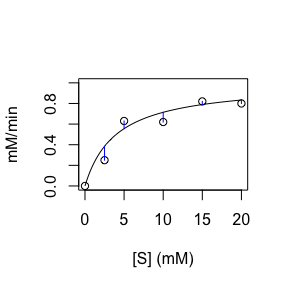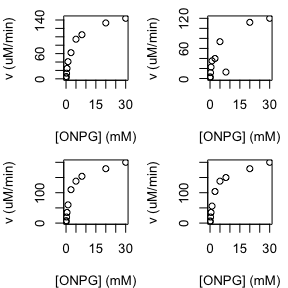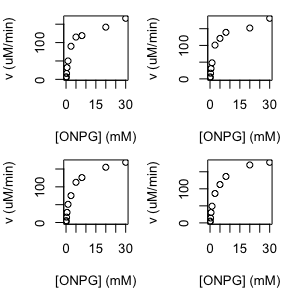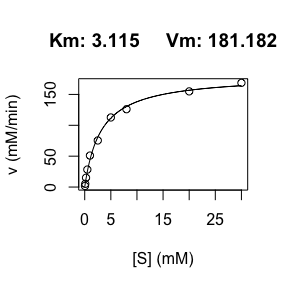# Fitting the Michaelis-Menten Model

library(renz)
data(ONPG)

## Non-linear least square

Biochemistry is an eminently quantitative science. The study of the relationship between variables and the determination of the parameters governing such relation, are part of the work of our discipline. Generally, the variables of interest are not linearly related to each other. Although exist linearizing transformations, these are not without risks since they can introduce significant biases.

The existence of programming languages such as R, allows us to easily and reliably address the non-linear fit of biochemical models. The function dir.MM() from the renz package carry out the non-linear least square fitting of kinetic data to the Michaelis-Menten equation.

$\begin{equation} \tag{1} v = V_{max} \frac{[S]}{K_m + [S]} \end{equation}$

Regression analysis is a set of statistical processes for estimating the relationships between a dependent variable (in our case initial rate) and one or more independent variables (in our case the substrate concentration). The method least squares computes the unique line (or hyperplane) that minimizes the sum of squared differences between the true data and that line (see Figure).$\begin{equation} \tag{2} SSQ = \sum_{i=1}^n[v_i - V_{max} \frac{[S_i]}{K_m + [S]_i}]^2 \end{equation}$

To find the kinetic parameters ($$K_m$$ and $$V_{max}$$) that minimize the SSQ, we will have to solve the system of equations:

$\begin{equation} \tag{3} \frac{\partial SSQ}{\partial V_{max}} = 0 = -2\sum_{i=1}^n[v_i - V_{max} \frac{[S]_i}{K_m + [S]_i}] \frac{[S]_i}{K_m + [S]_i} \end{equation}$

$\begin{equation} \tag{4} \frac{\partial SSQ}{\partial K_m} = 0 = -2\sum_{i=1}^n[v_i - V_{max} \frac{[S]_i}{K_m + [S]_i}] \frac{V_{max}[S]_i}{([S]_i + K_m)^2} \end{equation}$

To achieve such purpose, the function dir.MM() invokes numerical method well implemented into R.

We start by loading some kinetic data obtained by students during their undergraduate laboratory training. Using $$\beta$$-galactosidase as an enzyme model, the students assess the effect of the substrate o-nitrophenyl-$$\beta$$-D-galactopynaroside (ONPG) on the initial rate (doi: 10.1002/bmb.21522). The data obtained by eight different groups of students can be loaded just typing:

data <- ONPG
library(knitr)
kable(data)
ONPG v1 v2 v3 v4 v5 v6 v7 v8
0.05 2.26 1.29 0.004 0.004 0.004 0.003 1.77 2.98
0.10 5.48 3.33 0.008 0.007 0.007 0.006 5.20 5.20
0.25 13.40 11.80 0.020 0.020 0.016 0.017 15.04 14.38
0.50 24.70 22.80 0.035 0.035 0.032 0.031 28.31 30.30
1.00 40.90 35.20 0.060 0.056 0.050 0.048 50.98 48.99
2.50 62.30 39.90 0.110 0.104 0.090 0.101 75.42 86.25
5.00 94.30 73.50 0.138 0.138 0.115 0.121 112.68 112.57
8.00 105.00 12.90 0.154 0.150 0.119 0.139 126.06 136.24
20.00 133.00 112.00 0.179 0.179 0.142 0.152 154.93 169.97
30.00 144.00 120.00 0.200 0.200 0.166 0.181 168.75 177.71

The first column gives the ONPG concentrations in mM, and the remaining 8 columns correspond to the initial rates. Note that while groups 1, 2, 7 and 8 decided to express their rates as $$\mu$$M/min, the remaining groups opted by mM/min. This information can be confirmed by checking the attributes of data:

attributes(data)
#> $names #>  "ONPG" "v1" "v2" "v3" "v4" "v5" "v6" "v7" "v8" #> #>$row.names
#>    1  2  3  4  5  6  7  8  9 10
#>
#> $[ONPG] #>  "mM" #> #>$v3, v4, v5, v6
#>  "mM/min"
#>
#> $class #>  "data.frame" #> #>$v1, v2, v7, v8
#>  "uM/min"

Thus, before continuing we are going to express all the rates using the same units: $$\mu$$M/min:

data[ , 4:7] <- 1000 * data[ , 4:7]

## First thing first: scatter plot

I strongly insist to my students that when we have to analyze data, the first thing we must do is a scatter diagram, since this will give us a first impression about our data and will guide us on how to proceed with the analysis. To lead by example, we will carry out such diagrams.

The first four groups:

oldmar <- par()$mar oldmfrow <- par()$mfrow
par(mfrow = c(2, 2))
par(mar = c(4, 4,1,1))
for (i in 2:5){
plot(data$ONPG, data[, i], ty = 'p', ylab = 'v (uM/min)', xlab = '[ONPG] (mM)') }par(mar = oldmar) par(mfrow = oldmfrow) The next four groups: oldmar <- par()$mar
oldmfrow <- par()$mfrow par(mfrow = c(2, 2)) par(mar = c(4, 4,1,1)) for (i in 6:9){ plot(data$ONPG, data[, i],
ty = 'p', ylab = 'v (uM/min)', xlab = '[ONPG] (mM)')
}par(mar = oldmar)
par(mfrow = oldmfrow)

In general, the data does not provide us with any surprises. That is, the relationship between the dependent variable (initial rate) and the independent variable ([ONPG]) is what we expect: hyperbolic curve. An exception is the rate obtained by group 2 when [ONPG] = 8 mM, which is clearly an “outlier”. No problem! We will remove that point from further analysis to prevent it from introducing artifacts.

data$v2 <- NA ## Direct Michaelis-Menten fitting Using the data from group 7 to illustrate the use of the dir.MM() function: dir.MM(data[ , c(1,8)], unit_v = "mM/min")#>$parameters
#>      Km      Vm
#>   3.115 181.182
#>
#> \$data
#>        S      v   fitted_v
#> 1   0.05   1.77   2.862275
#> 2   0.10   5.20   5.635521
#> 3   0.25  15.04  13.460773
#> 4   0.50  28.31  25.059751
#> 5   1.00  50.98  44.029648
#> 6   2.50  75.42  80.668744
#> 7   5.00 112.68 111.634011
#> 8   8.00 126.06 130.405398
#> 9  20.00 154.93 156.765737
#> 10 30.00 168.75 164.138910

We propose to the reader, as an exercise, to compare these results with those obtained when we use data from the remaining groups.